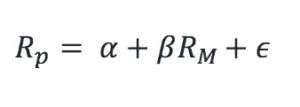# How to Calculate Systematic and Unsystematic Risk in STATA

Systematic risk is one the most discussed idea in the domain of corporate finance or financial risk management. It refers to the risk that effects the market at large or the whole market. Examples of risk that could effect large number of companies are economic or political instability, war, natural disaster. The other names used to refer to systematic risk are market risk, undiversifiable risk etc.  Unsystematic risk the exact opposite of systematic risk. It refers to the risk that may effect a single firm or small number of firms. That’s why it is also called firm-specific, unique or diversifiable risk. Examples of unsystematic risk could include fire in the factory, labor strike etc.

The aim of this article is not to explain the theoretical aspect of systematic and unsystematic risk, rather it is to present a step-by-step procedure on how to estimate systematic and unsystematic risk using STATA software.

#### Calculation of Systematic Risk

Before we calculate systematic risk, the first thing we need to understand is that systematic risk shows the sensitivity of  a stock return with respect to return on market. To measure this sensitivity, the beta coefficient from a regression model such as Capital Asset Pricing Model (CAPM) or Market Model can be used. To simply the learning process this article will use the Market Model. Following is the equation of market model:Rp stands for the return on a particular stock, where as Rm stands for the return on market. Click here to download the sample data in stata format or if you want to download the same data in csv format click here. So we want to estimate the beta of each stock in our dataset and store it. The stata command used for this purpose is called “statsby”. What statsby does is that it estimates the command across different cross-sections (which in our case are different stocks) and store its estimates in a stata file. We will use following command on our dataset:

statsby _b, by(symbol_code) saving(beta.dta) : regress stock_return market_return

Now lets first understand the command. We always start with the command so in this case the command that we are using is “statsby”. Next we write “_b” which means that we are asking stata to only store beta coefficients. After command the “by(symbol_code) tells stata to repeat the regression command (written at the end of statsby command. The next thing we need to tell stata the name of file that stata will create and save beta coefficient using “saving(beta.dta)” option. You can write any file name it is not mandatory to write “beta.dta”. The last part of the command is another command that will be repeated for every stock. In this case we are using simple OLS regression. So in short we are going to regress stock return on market return using the data of each stock and stock the beta coefficients in a separate file called beta.dta.

#### Calculation of Un-Systematic Risk

Unsystematic risk is also referred to as idiosyncratic risk in different research papers. The unsystematic risk is also estimated using the same above given equation but now instead of taking the beta coefficient, we need the error term, also called the residual. So after regressing the stock return on market return, the beta coefficient is used as a measure of systematic risk where as the residual from same equation is used as measure of unsystematic risk. You would be thinking that is quite simple, we just replace the “_b” with some “_e” in the statsby command and the rest command should remain same. But the bad new is that statsby command can save quite few types of statistics and residual is not one of them. Following commands are used to estimate unsystematic risk:

levelsof symbol_code, local(levels)

gen residual=.

foreach i of local levels {

regress stock_return market_return if symbol_code==`i’

predict temp, residuals

replace residual=temp if symbol_code==`i’

drop temp

}

egen idio_vola=sd(residual), by(symbol_code)

Before we explain the above code we need to understand that the standard deviation of residual for each stock is used as measure of unsystematic risk. It means that first we estimate the residual and then we take standard deviation of residual.

Subscribe
Notify of